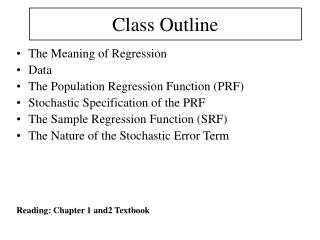# Class Outline - PowerPoint PPT PresentationDownload PresentationClass Outline

Class Outline
Download Presentation## Class Outline

- - - - - - - - - - - - - - - - - - - - - - - - - - - E N D - - - - - - - - - - - - - - - - - - - - - - - - - - -
##### Presentation Transcript

1. Class Outline • The Meaning of Regression • Data • The Population Regression Function (PRF) • Stochastic Specification of the PRF • The Sample Regression Function (SRF) • The Nature of the Stochastic Error Term Reading: Chapter 1 and2 Textbook

2. The Meaning of Regression Regression analysis is concerned with the study of the relationship between one variable called explained, or dependent, variable and one or more other variables called independent, or explanatory, variables. • Warning: Regression analysis does not imply causation. Causality between two or more variables should be determined on the basis of some theory.

3. The Meaning of Regression Statistical versus Deterministic Relationships We are concerned with what is known as the statistical, not functional or deterministic, dependence among variables. We deal with random or stochastic variables Regression versus Causation Regression does not imply causation. We need a theory to explain causation. Regression versus Correlation Correlation measure the strength or degree of linear association between two variables Regression estimates or predict the average value of one variable on the basis of the fixed values of other variables The dependent variable is assumed to be statistical, random, or stochastic. The explanatory variables are assumed to have fixed values

4. Data • Types of Data: Time Series, Cross Section, Pooled and Panel Data. • Sources of Data • Accuracy of Data

5. The Population Regression Function (PRF) Example: assume that we want to estimate the average consumption of 60 families in a community Population = 60 families We want to analyze the relationship between the Consumption expenditure of each family (Y) depending on the level of income (X)

6. The Population Regression Function (PRF)

7. The Population Regression Function (PRF) Graphically,

8. The Population Regression Function (PRF) • Population Regression Line (PRL) The PRL gives the average, or mean, value of the dependent variable corresponding to each value of the independent variable, in the population as a whole Since the PRL is approximately linear we can express it mathematically The PRL is a line that passes through the conditional means of Y. The mathematical equation is called Population Regression Function (PRF)

9. The Population Regression Function (PRF) • As a first approximation or a working hypothesis, we may assume that the PRF is a linear function of X Where 1 and 2 are the parameters of the model. By linear, we mean linearity on the parameters.

10. Stochastic Specification of the PRF We can express the deviation of an specific Yi around its expected value as Where the deviation ui is an unobservable random variable taking positive or negative values known as the stochastic disturbance (error term)

11. Stochastic Specification of the PRF This specification has two main parts: • Systematic or deterministic component • Nonsystematic component If we take the expected value of the PRF, we obtain the following

12. Significance of the Stochastic Disturbance Term The error term contains all the factors explained by other variables. Why not to include other variables? • Vagueness of theory • Unavailability of data • Core variables versus peripheral variables • Intrinsic randomness in human vehavior • Poor proxy variables • Principle of parsimony • Wrong functional form

13. The Sample Regression Function (SRF) • Most of the time we do not know the population • We only have a sample of this population • Different samples will provide different sets of information

14. The Nature of the Stochastic Error Term • Samples from our population

15. The Nature of the Stochastic Error Term • Mathematically, we can express this estimation as: where = estimator of E(Y/Xi) the estimator of the population conditional mean =estimator of 1 =estimator of 2

16. The Nature of the Stochastic Error Term Not all the sample data lie exactly on the respective sample regression line. Then, we need to develop the stochastic model, which we write as where = estimator of ui

17. The Nature of the Stochastic Error Term represents the difference between the actual Y values and their estimated values from the sample regression, that is In solving this estimation problem we do not observe 1, 2 and u. What we observe are their proxies